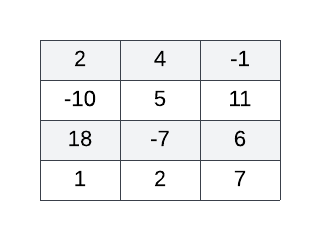Login or Create an Account to view the mark scheme, comment, and add to a test
Consider the following pseudocode:
VALUES = [ ] loop I from 0 to 3 VALUES[I] = ARRAY[I] loop J from 1 to 2 if ARR[I][J] < VALUES[I] then VALUES[I] = ARR[I][J] end if end loop end loop output VALUES
Choose the output yielded when the above algorithm is correctly applied to the 2D array given below:(a).
[4, 11, 18, 7]
(b).
[-1, -10, -7, 1]
(c).
[ -10, -7, -1]
(d).
[18, 5, 11]

HomeThe Right Test - Fast